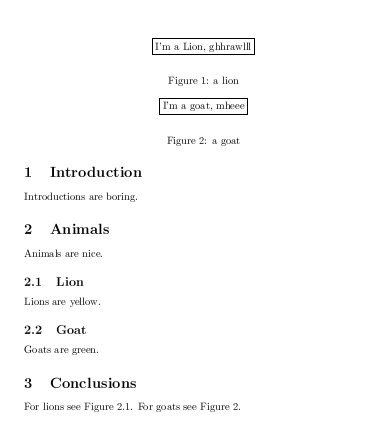The order of \caption and \label declarations matter in LaTeX floats (tables and figures). It is important to get references to the float right. The \label should come after the \caption or even inside the \caption environment. If you put the \label before the \caption you will get a reference to the (sub)section where float is declared instead of a reference to the float itself.

Consider the following LaTeX code:

\documentclass{article}

\begin{document}

\section{Introduction} %%%%%%%%%%%%%%%%%%%%%%%%%%
Introductions are boring.

\section{Animals} %%%%%%%%%%%%%%%%%%%%%%%%%%%%%%%
Animals are nice.

\subsection{Lion} % % % % % % % % % % % % % % % %
Lions are yellow.
\begin{figure}
\begin{center}\fbox{I'm a Lion, ghhrawlll}\end{center}
\label{fig:lion}\caption{a lion}
\end{figure}

\subsection{Goat} % % % % % % % % % % % % % % % %
Goats are green.
\begin{figure}
\begin{center}\fbox{I'm a goat, mheee}\end{center}
\caption{a goat}\label{fig:goat}
\end{figure}

\section{Conclusions} %%%%%%%%%%%%%%%%%%%%%%%%%%%
For lions see Figure~\ref{fig:lion}.
For goats see Figure~\ref{fig:goat}.

\end{document}


As you can see the lion figure has a \label before its \caption. The goat has it the other way around. Running it through latex gives the following result: